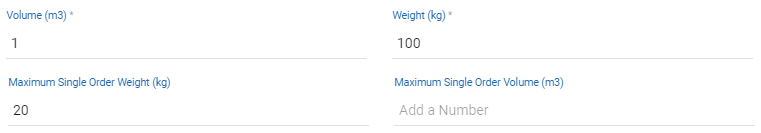# Vehicle Capacity Constraint Definitions

• Vehicle capacity constraint refers to:
• 1 - The maximum weight load and volume load that a vehicle can carry
• 2 - The maximum weight and volume of a single Order that a vehicle can carry

# Set Up Vehicle Capacity Constraint

• Navigate to Transportation > Vehicles tab
• Click Edit of the vehicle you wish to set up to open the Update Vehicle form
• On this form, the Weight (kg) and Volume (m3) fields are where you can set up the maximum weight load and volume load that vehicle can carry, while the Maximum Single Order Weight (kg) and Maximum Single Order Volume (m3) fields are where you can set up the maximum weight and volume of a single Order that vehicle can carry• Note: If you leave the Maximum Single Order Weight (kg) and Maximum Single Order Volume (m3) fields blank, the system will suppose that the maximum weight and volume of an Order that a vehicle can carry equal to the volume and weight capacity of that vehicle

# How Does This Work?

• During the Route Plan Optimization process, the system will compare the weight and volume of the Orders against the following attributes of the vehicles: 1. The weight and volume capacity of the vehicles; and 2. The maximum single Order weight and volume (If available) of the vehicles. There would be 3 scenarios:
• Scenario 1: The Order's weight and volume are smaller than both the weight and volume capacity and the maximum single Order weight and volume constraint of the vehicle. In this scenario, the vehicle can carry that Order
• Scenario 2: The Order's weight and volume are smaller than the weight and volume capacity but are bigger than the maximum single Order weight and volume constraint of the vehicle. In this scenario, the vehicle can not carry that Order
• Scenario 3: The Order's weight and volume are bigger than both the weight and volume capacity and the maximum single Order weight and volume constraint of the vehicle. In this scenario, the vehicle can not carry that Order